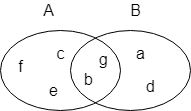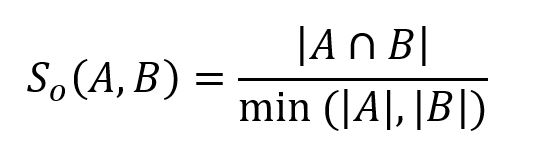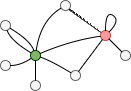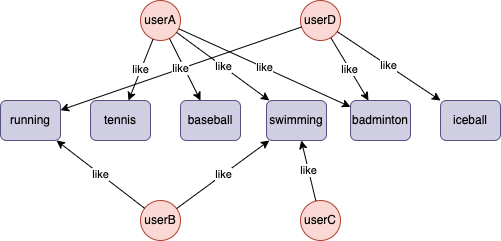# Change Nickname

Current Nickname:
Search
v4.0
v4.0

# Overlap Similarity

## Overview

Overlap similarity is derived from Jaccard similarity, which is also called the Szymkiewicz–Simpson coefficient. It divides the size of the intersection of two sets by the size of the smaller set with the purpose to indicate how similar the two sets are.

In application, elements in set typically are a series of properties of an entity. For instance, when calculating the similarity between two credit applications, elements are the phone number, email, device IP, company name and so on in the application form. In general graph applications, these kinds of information are often stored as properties of a node; however, when executing this algorithm, these information is designed as nodes and incorporated into the graph.

The range of values of overlap similarity is [0,1]; the larger the value, the more similar the two sets are.

## Basic Concept

### Set

A set consists of multiple elements; elements in a set are unordered and distinct; the number of elements in set A is the size of set A, denoted as `|A|`.

Set that consists of common elements of set A and set B is called the intersection of A and B, denoted as `A⋂B`.In the image above, set A is `{b,c,e,f,g}`, set B is `{a,d,b,g}`, intersection A⋂B is `{b,g}`.

### Overlap Similarity

Known sets A and B, overlap similarity between them can be expressed as:Overlap similarity between sets A and B in the previous example can be calculated upon this definition: `2 / 4 = 0.5`.

### Neighbors

In the graph, `Kx` is the set of neighbors of node `x` to represent set `A`, `Ky` is the set of neighbors of node `y` to represent set `B`. Note that neither `Kx` nor `Ky` contains repeated value, nor `x`, nor `y`, so the following interferences need to be eliminated when finding neighbors by edge in the graph:

• Multiple edges between `x`/`y` and their neighbors
• Self-loop edges of `x` and `y`
• Edges between `x` and `y`In the graph above, the red node has 3 neighbors (excludes the green node), the green node has 5 neighbors (excludes the red node), they have 2 common neighbors, their overlap similarity is `2 / 3 = 0.6667`.

## Special Case

### Lonely Node, Disconnected Graph

There is rarely computational valuable lonely node (empty set) in practice, intersection that involves lonely node is empty, and overlap similarity is 0.

For two nodes belong to different connected components, their overlap similarity must be 0.

### Self-loop Edge

Self-loop edge of a node does not increase the number of neighbors of the node.

### Directed Edge

For directed edges, the algorithm ignores the direction of edges but calculates them as undirected edges.

## Results and Statistics

The graph below shows which sports userA, userB, userC and userD (their UUID are 1, 2, 3 and 4 in order) likes:Algorithm results 1: Calculate the overlap similarity between each user and other 3 users, return `node` and `top_list`; `top_list` is all nodes which has the similarity > 0 with the node, those nodes' UUID and the similarity between then are displayed (ordered by the descending similarity)

node top_list
1 3:1.000000, 2:0.500000, 4:0.333333
2 3:1.000000, 4:0.500000, 1:0.500000
3 1:1.000000, 2:1.000000
4 2:0.500000, 1:0.333333

Algorithm results 2: Calculate the overlap similarity between userC and other 3 users, return `node1`, `node2` and `similarity`

node1 node2 similarity
3 1 1
3 2 1
3 4 0

Algorithm statistics: N/A

## Command and Configuration

• Command: `algo(similarity)`
• Configurations for the parameter `params()`:
Name
Type
Default
Specification
Description
ids / uuids []`_id` / []`_uuid` / Mandatory IDs or UUIDs of the first set of nodes to be calculated
ids2 / uuids2 []`_id` / []`_uuid` / Optional IDs or UUIDs of the second set of nodes to be calculated
type string cosine jaccard / overlap / cosine / pearson / euclideanDistance / euclidean Measurement of the similarity; jaccard means to calculate Jaccard similarity, overlap means to calcualte overlap similarity, cosine means to calcualte cosine similarity, pearson means to calculate Pearson correlation coefficient, euclideanDistance means to calculate Euclidean distance, euclidean means to calcualte normalzied Euclidean distance
limit int -1 >=-1 Number of results to return; return all results if sets to -1 or not set
top_limit int -1 -1 or >=0 Only available when ids2 and uuids2 are ignored, limit the length of selection results `top_list` of each node, return the full `top_list` if sets to -1 or not set

This algorithm has two calculation modes:

1. Selection mode

• When ids2 and uuids2 are ignored or given empty value, for each node in ids/uuids, select all nodes that have similarity > 0 with it (ordered by the descending similarity).
• The configuration item limit is to control the number of nodes in ids/uuids to return.

2. Pairing mode

• When ids2/uuids2 is given solid value, pair node in ids/uuids with node in ids2/uuids2 (Cartesian product), similarities are calculated for each node pair.
• The configuration item limit is to control the number of nodes pairs to return.

Example: Calculate overlap similarity between any two nodes in set A (UUID = 1,2) and set B (UUID = 3,4)

``````algo(similarity).params({
uuids: [1,2],
uuids2: [3,4],
type: "overlap"
}) as similarity
return similarity
``````

Example: For each node in set (UUID = 1,2,3), calculate the most similar node to each node measured by overlap similarity

``````algo(similarity).params({
uuids: [1,2,3],
type: "overlap",
top_limit: 1
}) as nodes
return nodes
``````

## Algorithm Execution

#### 1. File Writeback

Configuration
Data in Each Row
filename `node`,`top_list` or `node1`,`node2`,`similarity`

Example: Calculate overlap similarity between node UUID = 3 and other nodes, write the algorithm results back to file named s3

``````algo(similarity).params({
uuids: 3,
uuids2: [1,2,4],
type: "overlap"
}).write({
file:{
filename: "s3"
}
})
``````

#### 2. Property Writeback

Not supported by this algorithm.

#### 3. Statistics Writeback

This algorithm has no statistics.

### Direct Return

Alias Ordinal Type Description Column Name
0 []perNode / []perNodePair Node and its selection results / Node pair and its similarity `node`, `top_list` / `node1`, `node2`, `similarity`

Example: Calculate overlap similarity between each node and other nodes, define algorithm results as alias named similarity, and return the results

``````algo(similarity).params({
uuids: [1,2,3,4],
type: "overlap"
}) as similarity
return similarity
``````

### Streaming Return

Alias Ordinal Type Description Column Name
0 []perNode / []perNodePair Node and its selection results / Node pair and its similarity `node`, `top_list` / `node1`, `node2`, `similarity`

Example: Calculate overlap similarity between each node and other nodes, only keep the one with the highest similarity, define algorithm results as alias named similarity, and return the results

``````algo(similarity).params({
uuids: [1,2,3,4],
type: "overlap",
top_limit: 1
}).stream() as similarity
return similarity
``````

### Real-time Statistics

This algorithm has no statistics.